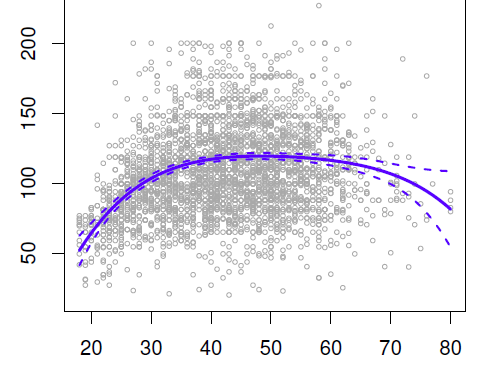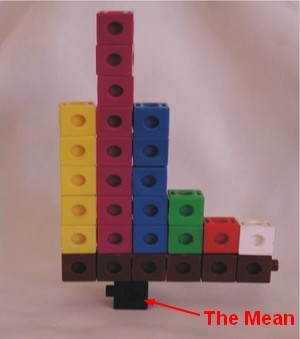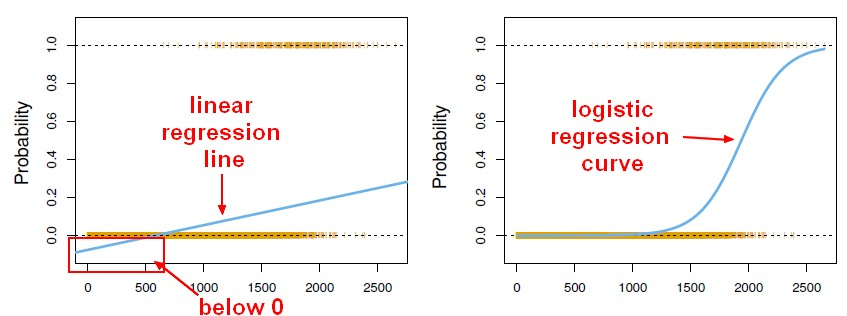# Statistics - (Estimator|Point Estimate) - Predicted (Score|Target|Outcome| )

An estimator or point estimate is a statistic that is used to infer the value of an unknown parameter in a statistical model.

A point is a value in this entire possible range of values from the distribution.

This Sample statistics are also called “point estimates” because they can take only just one point in an entire possible sampling distribution.

The hat on a statistics designs an estimator (ie means “estimated” from the equation that is build from the training data).
Example:

• $Y$ is the original target score from the training data (ie collected)
• $\hat{Y}$ is the predicted score from the model.

## Documentation / Reference

Recommended Pages(Machine|Statistical) Learning - (Target|Learned|Outcome|Dependent|Response) (Attribute|Variable) (Y|DV)

An (outcome|dependent) variable is ameasure that we want to predict. : the original score collected : the predicted score (or estimator) from the equation. The hat means “estimated” from the...Data Mining - (Global) Polynomial Regression (Degree)

polynomials regression Although polynomials are easy to think of, splines are much better behaved and more local. With polynomial regression, you create new variables that are just transformations...Data Mining - Root mean squared (Error|Deviation) (RMSE|RMSD)

Root mean squared (Error|Deviation) in case of regression. The RMSD represents the sample standard deviation of the differences between predicted values and observed values. The RMSE serves to aggregate...Distribution - (Mean|Average) (M| | )

The average is a measure of center that statisticians call the mean. To calculate the mean, you add all numbers and divide the total by the number of numbers (N). The mean is not resistant. The...Fuzzy Logic (Partial Truth)

Fuzzy logic is a form of many-valued logic that deals with approximate, rather than fixed and exact reasoning. Compared to traditional binary logic (where variables may take on true or false values),...Machine Learning - (Univariate|Simple) Logistic regression

A Simple Logistic regression is a Logistic regression with only one parameters. For the generalization (ie with more than one parameter), see Logistic regression comes from the fact that linear regression...Machine Learning - Logistic regression (Classification Algorithm)

The prediction from a logistic regression model can be interpreted as the probability that the label is 1. linear regression can also be used to perform classification problem. Just by transforming the...Model Building - ReSampling Validation

Resampling method are a class of methods that estimate the test error by holding out a subset of the training set from the fitting process, and then applying the statistical learning method to those held...R - (dependent|paired sample) t-test

(dependent|paired sample) t-test in R The Mean of differences is the point estimate The confidence interval is the interval estimate around the mean difference (not around the mean of the samples)...Statistics - (Confidence|likelihood) (Prediction probabilities|Probability classification)

Prediction probabilities are also known as: confidence (How confident can I be of this prediction?). or likelihood: (How likely is this prediction to be true?) They gives the probability of a predicted...